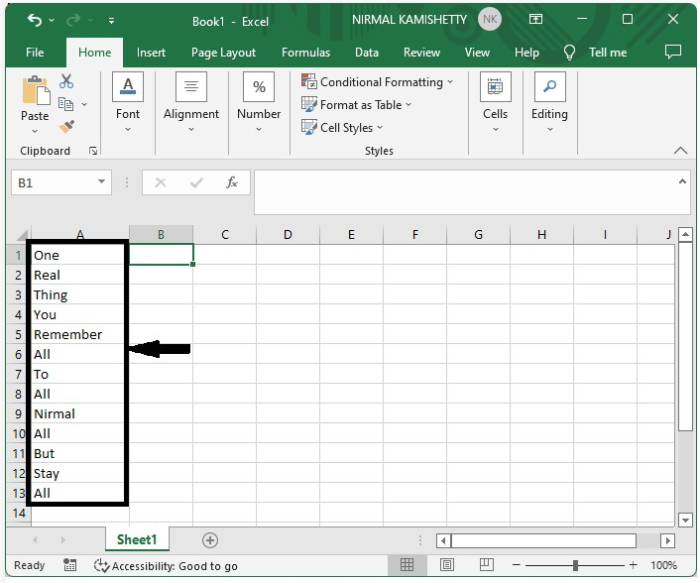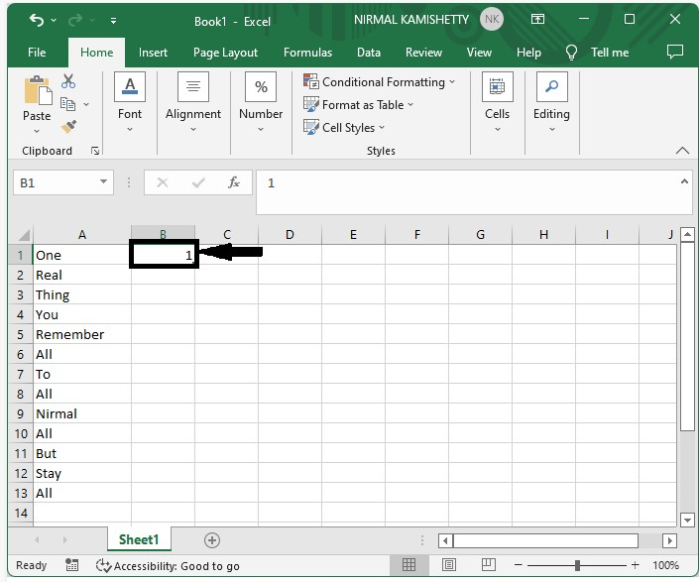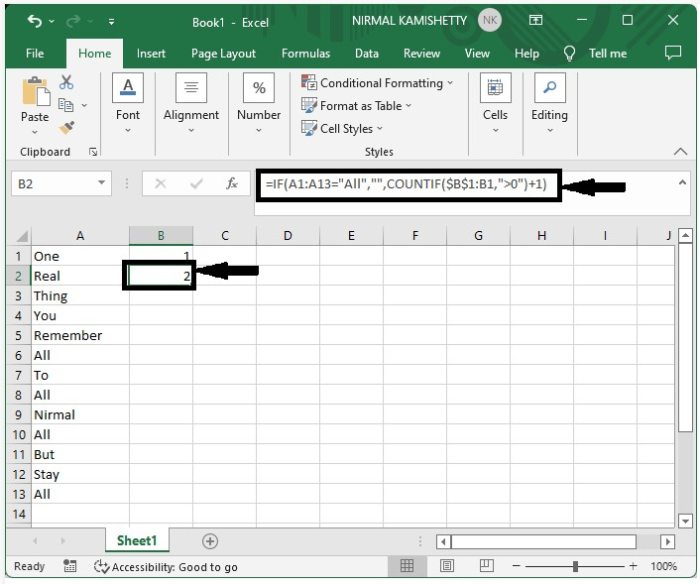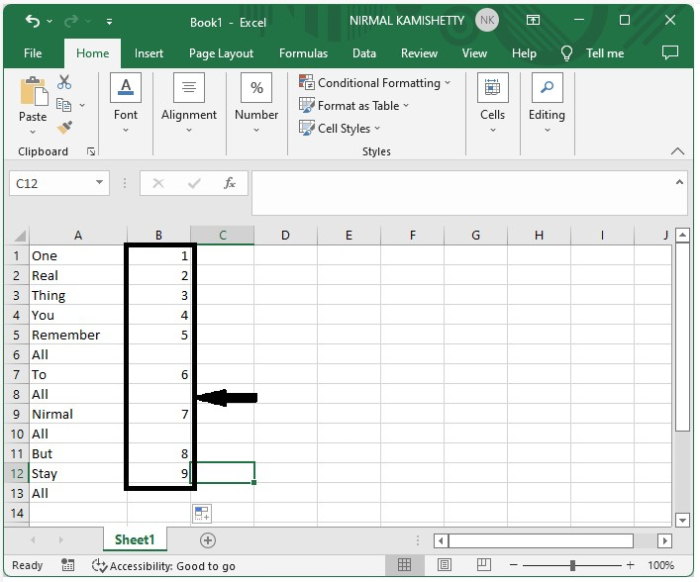# How to Auto-Number a Column Based on the Cell Values on Another Column in Excel?

You could have tried to auto number a column based on other column only based on uniqueness of the cell values but have you ever tried to auto number a column based on cell value on another column. This article will help you to understand how we can auto number a column based on cell values on other columns in excel.

Let us consider a situation where you want to count the no of items in a list excluding a single repeated and you don’t want to delete the item. This tutorial will help you to solve these kinds of problems in an efficient way.

## Auto-Number a Column Based on the Cell Values on Another Column

Here we will first assign one to first value the use the formula for the second value then use the auto fill handle to complete our task. Let us see a simple process to auto number a column based in cell value on another column in excel in a fast and efficient way.

Step 1

Let us consider a list of names of names as shown in below image and you want to calculate the total no of items excluding a single repeated name.Now click on the cell beside first result that is cell B1 and enter 1 in it. if the excluding item is present in cell A1 then enter the 1 in cell B2 as shown in below image.Step 2

Now click on the cell B2 and enter the formula as

=IF(A1:A13="All","",COUNTIF($B$1:B1,">0")+1) in the formula box and click Enter to get the first result as shown in below image.Step 3

As we got the first result to get the all the results drag down from the corner of cell B2 and our final output will be similar to the data shown in below image.## Conclusion

In this tutorial, we used a simple example to demonstrate how we can auto-number a column based on the cell values in another column in Excel.